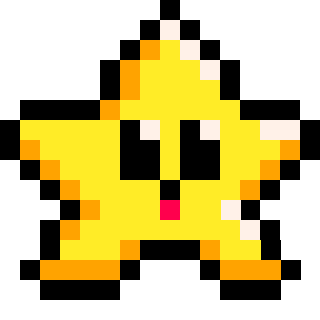# FOR

``````for variable=start, stop, [count_by] do
--code to repeat
end``````

 `variable` Often named as `i` to mean the "index" number of the loop. This number will indicate the current count of the loop. `start` A number, often set to `0` or `1` depending on how you might use the variable to keep track of the loop count and current index number. `stop` A number, used to end the loop when the index variable reaches beyond this stop number. `count by` (optional, default 1) A number, added to the index variable before the loop repeats. Negative numbers will decreas the index number.

"For loops" begin counting from the "start" number, count up or down by the "count by" number, and stops after reaching the "stop" number.

A "for loop" is a programming structure that allows you to repeat a set of instructions multiple times. It's like a machine that can do the same task over and over again. You tell the machines how many times to repeat the actions it is meant to do, and it does the rest. It's very useful for doing repetitive tasks quickly and efficiently.###### Examples:

``````for i=1, 9, 2 do
print( i )
end

--prints: 1 3 5 7 9``````

If the "count by" number is left out, then it will automatically be 1.

``````for i=1, 9 do
print( i )
end

--prints: 1 2 3 4 5 6 7 8 9``````

If you want to count down, then make the "count by" number a negative number.

``````for i=10, 3, -1 do
print( i )
end

--prints: 10 9 8 7 6 5 4 3``````

It's important to note that the index number is not checked until the end of each loop, so even when the "start" and "stop" numbers are the same, the loop will still run once through.

``````for i=0, 0 do
print( i )
end

--prints: 0``````

You may come across or need to use a double loop, or a loop within a loop. These are called "nested loops". Here is an example:

``````for i=0, 3 do
for j=1, 2 do
print( j )
end
print( "i="..i )
end

--prints: 1 2 i=0 1 2 i=1 1 2 i=2 1 2 i=3``````

You can differentiate the two loops by saying "outer" and "inner" loop. Just as it is common to use `i` as the index variable name, it is also common to then use the next letters, `j`, `k`, etc. for each inner loop. Notice that the index number doesn't change109

17 Mar 2023

`Font`